# Hopf Algebra Project

5474 words (22 pages) Essay

18th Sep 2017 Mathematics Reference this

Disclaimer: This work has been submitted by a university student. This is not an example of the work produced by our Essay Writing Service. You can view samples of our professional work here.

Any opinions, findings, conclusions or recommendations expressed in this material are those of the authors and do not necessarily reflect the views of UKEssays.com.

Petros Karayiannis

Chapter 0

## Introduction

Hopf algebras have lot of applications. At first, they used it in topology in 1940s, but then they realized it has applications through combinatorics, category theory, Hopf-Galois theory, quantum theory, Lie algebras, Homological algebra and functional analysis.

The purpose of this project is to see the definitions and properties of Hopf algebras.(Becca 2014)

## Preliminaries

This chapter provides all the essential tools to understand the structure of Hopf algebras. Basic notations of Hopf algebra are:

1. Groups
2. Fields
3. Vector spaces
4. Homomorphism
5. Commutative diagrams

## 1.Groups

Group G is a finite or infinite set of elements with a binary operation. Groups have to obey some rules, so we can define it as a group. Those are: closure, associative, there exist an identity element and an inverse element. Let us define two elements U, V in G, closure is when then the product of UV is also in G. Associative when the multiplication (UV) W=U (VW) ê“¯ U, V, W in G. There exist an identity element such that IU=UI=U for every element U in G. The inverse is when for each element U of G, the set contains an element V=U-1 such that UU-1=U-1U=I.

## 2.Fields

A field Òœ is a commutative ring and every element b Ïµ Òœ has an inverse.

## 3.Vector Space

A vector space V is a set that is closed under finite vector addition and scalar multiplication. In order for V to be a vector space, the following conditions must hold ê“¯ X, Y Ïµ V and any scalar a, b Ïµ Òœ:

• a(b X) = (a b) X
• (a + b) X=aX + bX
• a(X+Y)=aX + aY
• 1X=X

A left ideal of K-algebra is a linear subspace that has the property that any element of the subspace multiplied on the left by any element of the algebra produces an element of the subspace. We say that a subset L of a K-algebra A is a left ideal if for every x and y in L, z in A and c in K, we have the following:

1. X +y is in L
2. cx is in L
3. zâ‹… x is in L

If we replace c) with xâ‹… z is in L, then this would define a right ideal. A two-sided ideal is a subset that is both a left and a right ideal. When the algebra is commutative, then all of those notions of ideal are equivalent. We denote the left ideal as âŠ³.

## 4.Homomorphism

Given two groups, (G,*) and (H,Â°) is a function f: Gâ†’H such that ê“¯ u, v Ïµ G it holds that

f(u*v)=f(u)Â°f(v)

## 5.Commutative diagrams

A commutative diagram is showing the composition of maps represented by arrows.

The fundament operation of Hopf algebras is the tensor product. A tensor product is a multiplication of vector spaces V and W with a result a single vector space, denoted as V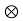Â  W.

## Definition 0.1

Let V and W be Òœ-vector spaces with bases {ei } and {fj } respectively. The tensor product V and W is a new Òœ-vector space,Â Â  VÂÂ  W with basis { eifj }, is the set of all elements vÂ  w= âˆ‘ (ci,j eiÂ  fj ). ci,j ÏµÒœ are scalars.

Also tensor products obey to distributive and scalar multiplication laws.

The dimension of the tensor product of two vector spaces is:

Dim(VÂ  W)=dim(V)dim(W)

## Theorem of Universal Property of Tensor products 0.2

Let V, W, U be vector spaces with map f: V x W â†’ U is defined as f: (v, w) â†’vw.

1. There exists a bilinear mapping b: V x W â†’ VÂ  W , (v,w) â†’ vÂ Â  w
2. If f: V x W â†’ U is bilinear, then there exist a unique function, f: VÂ  Wâ†’U with f=fÂ°b

## Â Extension of Tensor Products0.3

The definition of Tensor products can be extended for more than two vectors such as;

V1 âŠ-Â  V2âŠ-Â Â  V3 âŠ-Â  â€¦..âŠ-Â  VN = âˆ‘( biv1âŠ-Â  v2âŠ-Â  â€¦.âŠ-Â  vn )Â  (Becca 2014)

## Definition0.4

Let U,V be vector spacers over a field k and Î½ Ïµ Uâ¨‚V. If Î½=0 then Rank (Î½) =0. If Î½â‰ 0 then rank (Î½) is equal to the smallest positive integer r arising from the representations of Î½= âˆ‘ui â¨‚ vi ÏµUâ¨‚V for i=1,2,â€¦,r.

## Definition0.5

Let U be a finite dimensional vector space over the field k with basis {u1,â€¦.,un}Â  be a basis for U. the dual basis for U*is {u1,â€¦.,un} where ui(uj)= Î´ij for 1â‰¤I,jâ‰¤n.

## Dual Pair0.6

A dual pair is a 3 -tuple (X,Y,<,>) consisting two vector spaces X,Y over the same field K and a bilinear map, < , >: X x Yâ†’K with ê“¯x Ïµ X{0}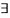yÏµY: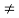0 and ê“¯y Ïµ Y{0}xÏµX:0

## Definition0.7

The wedge product is the product in an exterior algebra. If Î±, Î² are differential k-forms of degree p, g respectively, then

Â Î±âˆ§Î²=(-1)pq Î²âˆ§Î±, is not in general commutative, but is associative,

(Î±âˆ§Î²)âˆ§u= Î±âˆ§(Î²âˆ§u) and bilinear

(c1 Î±1+c2 Î±2)âˆ§ Î²= c1( Î±1âˆ§ Î²) + c2( Î±2âˆ§ Î²)

Î±âˆ§( c1 Î²1+c2 Î²2)= c1( Î±âˆ§ Î²1) + c2( Î±âˆ§ Î²2).Â Â Â  (Becca 2014)

## Definition1.1

“Let (A, m, Î·) be an algebra over k and write mop (a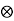b) = a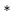b ê“¯ a, bÏµ A where mop=m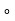Ï„Î‘,Î‘. Thus ab=ba ê“¯a, b ÏµA. The (A, mop, Î·) is the opposite algebra.”

## Definition1.2

“A co-algebra C is

1. A vector space over K
2. A map Î”: Câ†’C âŠ-Â  C which is coassociative in the sense of âˆ‘ (c(1)(1) âŠ-Â Â  c(1)(2) âŠ-Â  c(2))= âˆ‘ (c(1) âŠ-Â Â  c(2)(1) âŠ-Â  c(2)c(2) )Â Â  ê“¯ cÏµC (Î” called the co-product)
3. A map Îµ: Câ†’ k obeying âˆ‘[Îµ((c(1))c(2))]=c= âˆ‘[(c(1)) Îµc(2))] ê“¯ cÏµC ( Îµ called the counit)”

Co-associativity and co-unit element can be expressed as commutative diagrams as follow: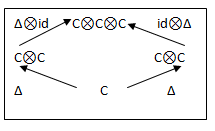Figure 1: Co-associativity map Î”Figure 2: co-unit element map Îµ

## Definition1.3“A bi-algebra H is

1. An algebra (H, m ,Î·)
2. A co-algebra (H, Î”, Îµ)
3. Î”,Îµ are algebra maps, where HâŠ-Â  H has the tensor product algebra structure (hâŠ- g)(hâŠ-Â  g)= hhâŠ-Â Â  gg ê“¯h, h, g, g ÏµH. “

A representation of Hopf algebras as diagrams is the following: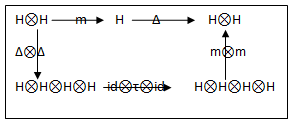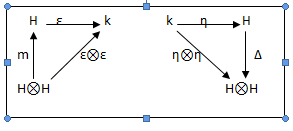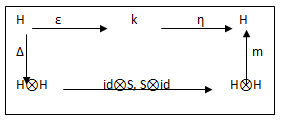## Definition1.4

“A Hopf Algebra H is

1. A bi-algebra H, Î”, Îµ, m, Î·
2. A map S : Hâ†’ H such that âˆ‘ [(Sh(1))h(2) ]= Îµ(h)= âˆ‘ [h(1)Sh(2) ]ê“¯ hÏµH

The axioms that make a simultaneous algebra and co-algebra into Hopf algebra is

Ï„:Â  HâŠ- Hâ†’HâŠ-H

Is the map Ï„(hâŠ-g)=gâŠ-h called the flip map ê“¯ h, g Ïµ H.”

## Definition1.5

“Hopf Algebra is commutative if it’s commutative as algebra. It is co-commutative if it’s co-commutative as a co-algebra, Ï„Î”=Î”. It can be defined as S2=id.

A commutative algebra over K is an algebra (A, m, Î·) over k such that m=mop.”

## Definition1.6

“Two Hopf algebras H,H are dually paired by a map < , > : H’H â†’k if,

<Ï†Ïˆ, h> =<Ï†Ïˆ,Î”h>, <1,h>=Îµ(h)

<Î”Ï†, hgÂ  >=<Ï†, hg>, Îµ(Ï†)=<Ï†,1>

=<Ï†, Sh>

ê“¯ Ï†, ÏˆÏµ H’ and h, g ÏµH.

Let (C, Î”,Îµ) be a co-algebra over k. The co-algebra (C, Î”cop, Îµ) is the opposite co-algebra.

A co-commutative co-algebra over k is a co-algebra (C, Î”, Îµ) over k such that Î”= Î”cop.”

## Definition1.7

“A bi-algebra or Hopf algebra H acts on algebra A (called H-module algebra) if:

1. H acts on A as a vector space.
2. The product map m: AAâ†’A commutes with the action of H
3. The unit map Î·: kâ†’ A commutes with the action of H.

From b,c we come to the next action

hâŠ³(ab)=âˆ‘(h(1)âŠ³a)(h(2)âŠ³b), hâŠ³1= Îµ(h)1, ê“¯a, b Ïµ A, h Ïµ H

This is the left action.”

## Definition1.8

“Let (A, m, Î·) be algebra over k and is a left H- module along with a linear map m: AâŠ-Aâ†’A and a scalar multiplication Î·: k âŠ- Aâ†’A if the following diagrams commute.”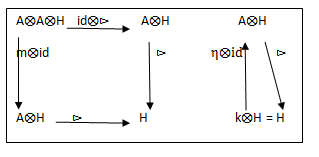Figure 3: Left Module map

## Definition1.9

“Co-algebra (C, Î”, Îµ) is H-module co-algebra if:

1. C is an H-module
2. Î”: Câ†’CC and Îµ: Câ†’ k commutes with the action of H. (Is a right C- co-module).

Explicitly,

Î”(hâŠ³c)=âˆ‘h(1)âŠ³c(1)â¨‚h(2)âŠ³c(2), Îµ(hâŠ³c)= Îµ(h)Îµ(c), ê“¯h Ïµ H, c Ïµ C.”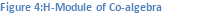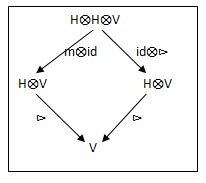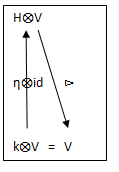## Â Definition1.10

“A co-action of a co-algebra C on a vector space V is a map Î²: Vâ†’Câ¨‚V such that,

1. (idâ¨‚Î²) âˆ˜Î²=(Î”â¨‚ id )Î²;
2. Â id =(Îµâ¨‚id )âˆ˜Î².”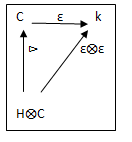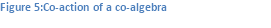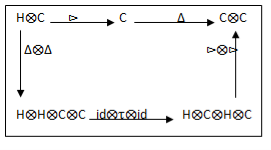## Definition1.11

“A bi-algebra or Hopf algebra H co-acts on an algebra A (an H- co-module algebra) if:

1. A is an H- co-module
2. The co-action Î²: Aâ†’ Hâ¨‚A is an algebra homomorphism, where Hâ¨‚A has the tensor product algebra structure.”

## Definition1.12

“Let C be co- algebra (C, Î”, Îµ), map Î²: Aâ†’ Hâ¨‚A is a right C- co- module if the following diagrams commute.”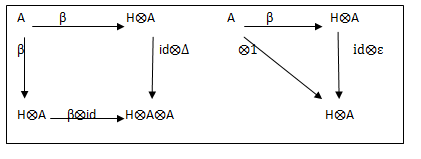Figure 6:Co-algebra of a right co-module

“Sub-algebras, left ideals and right ideals of algebra have dual counter-parts in co-algebras. Let (A, m, Î·) be algebra over k and suppose that V is a left ideal of A. Then m(Aâ¨‚V)âŠ†V. Thus the restriction of m to Aâ¨‚V determines a map Aâ¨‚Vâ†’V. Left co-ideal of a co-algebra C is a subspace V of C such that the co-product Î” restricts to a map Vâ†’Câ¨‚V.”

## Definition1.13

“Let V be a subspace of a co-algebra C over k. Then V is a sub-co-algebra of C if Î”(V)âŠ†Vâ¨‚V, for left co-ideal Î”(V)âŠ†Câ¨‚V and for right co-ideal Î”(V)âŠ†Vâ¨‚C.”

## Definition1.14

“Let V be a subspace of a co-algebra C over k. The unique minimal sub-co-algebra of C which contains V is the sub-co-algebra of C generated by V.”

## Definition1.15

“A simple co-algebra is a co-algebra which has two sub-co-algebras.”

## Definition1.16

“Let C be co-algebra over k. A group-like element of C is c ÏµC with satisfies, Î”(s)=sâ¨‚sÂ  and Îµ(s)=1 ê“¯ s ÏµS. The set of group-like elements of C is denoted G(C).”

## Definition1.17

“Let S be a set. The co-algebra k[S] has a co-algebra structure determined by

Î”(s)=sâ¨‚sÂ  and Îµ(s)=1

ê“¯ s ÏµS. If S=âˆ… we set C=k[âˆ…]=0.

Is the group-like co-algebra of S over k.”

## Definition1.18

“The co-algebra C over k with basis {co, c1, c2,â€¦..} whose co-product and co-unit is satisfy by Î”(cn)= âˆ‘cn-lâ¨‚cl and Îµ(cn)=Î´n,0 for l=1,â€¦.,n and for all nâ‰¥0. Is denoted by Pâˆž(k). The sub-co-algebra which is the span of co, c1, c2,â€¦,cn is denoted Pn(k).”

## Definition1.19

“A co-matrix co-algebra over k is a co-algebra over k isomorphic to Cs(k) for some finite set S. The co-matrix identities are:

1. Î”(ei, j)= âˆ‘ei, lâ¨‚el, j
2. Îµ(ei, j)=Î´i, j

âˆ€ i, j ÏµS. Set Câˆ…(k)=(0).”

## Definition1.20

“Let S be a non-empty finite set. A standard basis for Cs(k) is a basis {c i ,j}I, j ÏµS for Cs(k) which satisfies the co-matrix identities.”

## Definition1.21

“Let (C, Î”c, Îµc) and (D, Î”D, ÎµD) be co-algebras over the field k. A co-algebra map f: Câ†’D is a linear map of underlying vector spaces such that Î”Dâˆ˜f=(fâ¨‚f)âˆ˜ Î”c and ÎµDâˆ˜f= Îµc. An isomorphism of co-algebras is a co-algebra map which is a linear isomorphism.”

## Definition1.22

“Let C be co-algebra over the field k. A co-ideal of C is a subspace I of C such that Îµ (I) = (0) and Î” (Î™) âŠ† Iâ¨‚C+Câ¨‚I.”

## Definition1.23

“The co-ideal Ker (Îµ) of a co-algebra C over k is denoted by C+.”

## Definition1.24

“Let I be a co-ideal of co-algebra C over k. The unique co-algebra structure on C /I such that the projection Ï€: Câ†’ C/I is a co-algebra map, is the quotient co-algebra structure on C/I.”

Our academic experts are ready and waiting to assist with any writing project you may have. From simple essay plans, through to full dissertations, you can guarantee we have a service perfectly matched to your needs.

## Definition1.25

“The tensor product of co-algebra has a natural co-algebra structure as the tensor product of vector space CâŠ-D is a co-algebra over k where Î”(c(1)â¨‚d(1))â¨‚( c(2)â¨‚d(2)) and Îµ(câ¨‚d)=Îµ(c)Îµ(d) âˆ€ c in C and d in D.”

## Definition1.26

“Let C be co-algebra over k. A skew-primitive element of C is a cÏµC which satisfies Î”(c)= gâ¨‚c +câ¨‚h, where c, h ÏµG(c). The set of g:h-skew primitive elements of C is denotedÂ  by

Pg,h (C).”

## Definition1.27

“Let C be co-algebra over a field k. A co-commutative element of C is cÏµC such that Î”(c) = Î”cop(c). The set of co-commutative elements of C is denoted by Cc(C).

Cc(C) âŠ†C.”

## Definition1.28

“The category whose objects are co-algebras over k and whose morphisms are co-algebra maps under function composition is denoted by k-Coalg.”

## Definition1.29

“The category whose objects are algebras over k and whose morphisms are co-algebra maps under function composition is denoted by k-Alg.”

## Definition1.30

“Let (C, Î”, Îµ) be co-algebra over k. The algebra (Câˆ-, m, Î·) where m= Î”âˆ-| Câˆ-â¨‚Câˆ-, Î· (1) =Îµ, is the dual algebra of (C, Î”, Îµ).”

## Definition1.31

“Let A be algebra over the field k. A locally finite A-module is an A-module M whose finitely generated sub-modules are finite-dimensional. The left and right Câˆ--module actions on C are locally finite.”

## Definition1.32

“Let A be algebra over the field k. A derivation of A is a linear endomorphism F of A such that F (ab) =F (a) b-aF(b) for all a, b ÏµA.

For fixed b ÏµA note that F: Aâ†’A defined by F(a)=[a, b]= ab- baÂ  for all a ÏµA is a derivation of A.”

## Definition1.33

“Let C be co-algebra over the field k. A co-derivation of C is a linear endomorphism f of C such that Î”âˆ˜f= (fâ¨‚IC + IC â¨‚f) âˆ˜Î”.”

## Definition1.34

“Let A and B ne algebra over the field k. The tensor product algebra structure on Aâ¨‚B is determined by (aâ¨‚b)(a’â¨‚b’)= aa’â¨‚bb’ ê“¯ a, a’ÏµA and b, b’ÏµB.”

## Definition1.35

“Let X, Y be non-empty subsets of an algebra A over the field k. The centralizer of Y in X is

ZX(Y) = {xÏµX|yx=xy ê“¯yÏµY}

For y ÏµA the centralizer of y in X is ZX(y) = ZX({y}).”

## Definition1.36

“The centre of an algebra A over the field Z (A) = ZA(A).”

## Definition1.37

“Let (S, â‰¤) be a partially ordered set which is locally finite, meaning that ê“¯, I, jÏµS which satisfy iâ‰¤j the interval [i, j] = {lÏµS|iâ‰¤lâ‰¤j} is a finite set. Let S= {[i, j] |I, jÏµS, iâ‰¤j} and let A be the algebra which is the vector space of functions f: Sâ†’k under point wise operations whose product is given by

(fâ‹†g)([i, j])=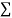f([i, l])g([l, j])Â  iâ‰¤lâ‰¤j

For all f, g ÏµA and [i, j]ÏµS and whose unit is given by 1([I,j])= Î´i,j ê“¯[I,j]ÏµS.”

## Definition1.38

“The algebra of A over the k described above is the incidence algebra of the locally finite partially ordered set (S, â‰¤).”

## Definition1.39

“Lie co-algebra over k is a pair (C, Î´), where C is a vector space over k and Î´: Câ†’Câ¨‚C is a linear map, which satisfies:

Ï„âˆ˜Î´=0 and (Î™+(Ï„â¨‚Î™)âˆ˜(Î™â¨‚Ï„)+(Î™â¨‚Ï„)âˆ˜ (Ï„â¨‚Î™))âˆ˜(Î™â¨‚Î´)âˆ˜Î´=0

Ï„=Ï„C,C and I is the appropriate identity map.”

## Definition1.40

“Suppose that C is co-algebra over the field k. The wedge product of subspaces U and V is Uâˆ§V = Î”-1(Uâ¨‚C+ Câ¨‚V).”

## Definition1.41

“Let C be co-algebra over the field k. A saturated sub-co-algebra of C is a sub-co-algebra D of C such that Uâˆ§VâŠ†D, ê“¯ U, V of D.”

## Definition1.42

“Let C be co-algebra over k and (N, Ï) be a left co-module. Then Uâˆ§X= Ï-1(Uâ¨‚N+ Câ¨‚X) is the wedge product of subspaces U of C and X of N.”

## Definition1.43

“Let C be co-algebra over k and U be a subspace of C. The unique minimal saturated sub-co-algebra of C containing U is the saturated closure of U in C.”

## Definition1.44

“Let (A, m, Î·) be algebra over k. Then,

1. Aâˆ˜=mâˆ–1(Aâˆ-â¨‚Aâˆ- )
2. (Aâˆ˜, Î”, Îµ) is a co-algebra over k, where Î”= mâˆ-| Aâˆ˜ and Îµ=Î·âˆ-.

Î¤he co-algebra (Aâˆ˜, Î”, Îµ) is the dual co-algebra of (A, m, Î·).

Also we denote Aâˆ˜ by aâˆ˜ and Î”âˆ˜= aâˆ˜(1)â¨‚ aâˆ˜(2), ê“¯ aâˆ˜ Ïµ Aâˆ˜.”

## Definition1.45

“Let A be algebra over k. An Î·:Î¾- derivation of A is a linear map f: Aâ†’k which satisfies f(ab)= Î·(a)f(b)+f(a) Î¾(b), ê“¯ a, bÏµ A and Î·, Î¾ Ïµ Alg(A, k).”

## Definition1.46

“The full subcategory of k-Alg (respectively of k-Co-alg) whose objects are finite dimensional algebras (respectively co-algebras) over k is denoted k-Alg fd (respectivelyÂ Â Â Â  k-Co-alg fd).”

## Definition1.47

“A proper algebra over k is an algebra over k such that the intersection of the co-finite ideals of A is (0), or equivalently the algebra map jA:Aâ†’(Aâˆ˜)*, be linear map defined by jA(a)(aâˆ˜)=aâˆ˜(a), a ÏµA and aâˆ˜ÏµAâˆ˜. Then:

1. jA:Aâ†’(Aâˆ˜)* is an algebra map
2. Ker(jA) is the intersection of the co-finite ideals of A
3. Im(jA) is a dense subspace of (Aâˆ˜)*.

Is one-to-one.”

## Definition1.48

“Let A (respectively C) be an algebra (respectively co-algebra ) over k. Then A (respectively C) is reflexive if jA:Aâ†’(Aâˆ˜)*, as defined before and jC:Câ†’(C*)âˆ˜, defined as:

jC(c)(c*)=c*(c), ê“¯ c*ÏµC* and cÏµC. Then:

1. Im(jC)âŠ†(C*)âˆ˜ and jC:Câ†’(C*)âˆ˜ is a co-algebra map.
2. jC is one-to-one.
3. Im(jC) is the set of all aÏµ(C*)* which vanish on a closed co-finite ideal of C*.

Is an isomorphism.”

## Definition1.49

“Almost left noetherian algebra over k is an algebra over k whose co-finite left ideal are finitely generated. (M is called almost noetherian if every co-finite submodule of M is finitely generated).”

## Definition1.50

“Let f:Uâ†’V be a map of vector spaces over k. Then f is an almost one-to-one linear map if ker(f) is finite-dimensional, f is an almost onto linear map if Im(f) is co-finite subspace of V and f is an almost isomorphism if f is an almost one-to-one and an almost linear map.”

## Definition1.51

“Let A be algebra over k and C be co-algebra over k. A pairing of A and C is a bilinear map

Â Î²: AÃ-Câ†’k which satisfies, Î²(ab,c)= Î² (a, c(1))Î² (b, c(2)) and Î²(1, c) = Îµ(c), ê“¯ a, b Ïµ A andÂ Â Â Â Â Â Â  c ÏµC.”

## Definition1.52

“Let V be a vector space over k. A co-free co-algebra on V is a pair (Ï€, Tco(V)) such that:

1. Tco(V) is a co-algebra over k and Ï€: Tco(V)â†’T is a linear map.
2. If C is a co-algebra over k and f:Câ†’V is a linear map,âˆƒ a co-algebra map F: Câ†’ Tco(V) determined by Ï€âˆ˜F=f.”

## Definition1.53

“Let V be a vector space over k. A co-free co-commutative co-algebra on V is any pair (Ï€, C(V)) which satisfies:

1. C(V) is a co-commutative co-algebra over k and Ï€:C(V)â†’V is a linear map.
2. If C is a co-commutative co-algebra over k and f: Câ†’V is linear map, âˆƒ co-algebra map F:C â†’C(V) determined by Ï€âˆ˜F=f. “Â Â Â Â Â Â Â Â Â Â Â Â Â Â Â Â Â  (Majid 2002, Radford David E)

## Proposition (Anti-homomorphism property of antipodes) 2.1

“The antipode of a Hopf algebra is unique and obey S(hg)=S(g)S(h), S(1)=1 and (Sâ¨‚S)âˆ˜Î”h=Ï„âˆ˜Î”âˆ˜Sh, ÎµSh=Îµh, âˆ€h,g âˆˆ H. “Â Â Â Â Â Â Â Â Â Â Â Â  (Majid 2002, Radford David E)

## Proof

Let S and S1 be two antipodes for H. Then using properties of antipode, associativity of Ï„ and co-associativity of Î” we get

S= Ï„âˆ˜(SâŠ-[ Ï„âˆ˜(IdâŠ-S1)âˆ˜Î”])âˆ˜Î”= Ï„âˆ˜(IdâŠ- Ï„)âˆ˜(Sâ¨‚IdâŠ-S1)âˆ˜(Id âŠ-Î”)âˆ˜Î”=

Ï„âˆ˜(Ï„â¨‚Id)âˆ˜(Sâ¨‚IdâŠ-S1)âˆ˜(Î” âŠ-Id)âˆ˜Î” = Ï„âˆ˜( [Ï„âˆ˜(Sâ¨‚Id)âˆ˜Î”]â¨‚S1)âˆ˜ Î”=S1.

So the antipode is unique.

Let Sâˆ-id=Îµs idâˆ-S=Îµt

To check that S is an algebra anti-homomorphism, we compute

S(1)= S(1(1))1(2)S(1(3))= S(1(1)) Îµt (1(2))= Îµs(1)=1,

S(hg)=S(h(1)g(1)) Îµt(h(2)g(2))= S(h(1)g(1))h(2) Îµt(g(2))S(h(3))=Îµs (h(1)g(1))S(g(2))S(h(2))=

S(g(1)) Îµs(h(1)) Îµt (g(2))S(h(2))=S(g)S(h), âˆ€h,g âˆˆH and we used Îµt(hg)= Îµt(h Îµt(g)) and Îµs(hg)= Îµt(Îµs(h)g).

Dualizing the above we can show that S is also a co-algebra anti-homomorphism:

Îµ(S(h))= Îµ(S(h(1) Îµt(h(2)))= Îµ(S(h(1)h(2))= Îµ(Îµt(h))= Îµ(h),

Î”(S(h))= Î”(S(h(1) Îµt(h(2)))= Î”(S(h(1) Îµt(h(2))â¨‚1)= Î”(S(h(1) ))(h(2)S(h(4))â¨‚ Îµt (h(3))=

Î”(Îµs(h(1))(S(h(3))â¨‚S(h(2)))=S(h(3))â¨‚ Îµs(h(1))S(h(2))=S(h(2))â¨‚ S(h(1)). (New directions)

## Example2.2

“The Hopf Algebra H=Uq(b+) is generated by 1 and the elements X,g,g-1 with relations

gg-1=1=g-1g and g X=q X g, where qÂ  is a fixed invertible element of the field k. Here

Î”X= Xâ¨‚1 +g â¨‚ X, Î”g=g â¨‚ g, Î”g-1=g-1â¨‚g-1,

ÎµX=0, Îµg=1=Îµ g-1, SX=- g-1X, Sg= g-1, S g-1=g.

S2X=q-1X.”

## Proof

We have Î”, Îµ on the generators and extended them multiplicatively to products of the generators.

Î”gX=(Î”g)( Î”X)=( gâ¨‚g)( Xâ¨‚1 +gâ

Petros Karayiannis

Chapter 0

## Introduction

Hopf algebras have lot of applications. At first, they used it in topology in 1940s, but then they realized it has applications through combinatorics, category theory, Hopf-Galois theory, quantum theory, Lie algebras, Homological algebra and functional analysis.

The purpose of this project is to see the definitions and properties of Hopf algebras.(Becca 2014)

## Preliminaries

This chapter provides all the essential tools to understand the structure of Hopf algebras. Basic notations of Hopf algebra are:

1. Groups
2. Fields
3. Vector spaces
4. Homomorphism
5. Commutative diagrams

## 1.Groups

Group G is a finite or infinite set of elements with a binary operation. Groups have to obey some rules, so we can define it as a group. Those are: closure, associative, there exist an identity element and an inverse element. Let us define two elements U, V in G, closure is when then the product of UV is also in G. Associative when the multiplication (UV) W=U (VW) ê“¯ U, V, W in G. There exist an identity element such that IU=UI=U for every element U in G. The inverse is when for each element U of G, the set contains an element V=U-1 such that UU-1=U-1U=I.

## 2.Fields

A field Òœ is a commutative ring and every element b Ïµ Òœ has an inverse.

## 3.Vector Space

A vector space V is a set that is closed under finite vector addition and scalar multiplication. In order for V to be a vector space, the following conditions must hold ê“¯ X, Y Ïµ V and any scalar a, b Ïµ Òœ:

• a(b X) = (a b) X
• (a + b) X=aX + bX
• a(X+Y)=aX + aY
• 1X=X

A left ideal of K-algebra is a linear subspace that has the property that any element of the subspace multiplied on the left by any element of the algebra produces an element of the subspace. We say that a subset L of a K-algebra A is a left ideal if for every x and y in L, z in A and c in K, we have the following:

1. X +y is in L
2. cx is in L
3. zâ‹… x is in L

If we replace c) with xâ‹… z is in L, then this would define a right ideal. A two-sided ideal is a subset that is both a left and a right ideal. When the algebra is commutative, then all of those notions of ideal are equivalent. We denote the left ideal as âŠ³.

## 4.Homomorphism

Given two groups, (G,*) and (H,Â°) is a function f: Gâ†’H such that ê“¯ u, v Ïµ G it holds that

f(u*v)=f(u)Â°f(v)

## 5.Commutative diagrams

A commutative diagram is showing the composition of maps represented by arrows.

The fundament operation of Hopf algebras is the tensor product. A tensor product is a multiplication of vector spaces V and W with a result a single vector space, denoted as VÂ  W.

## Definition 0.1

Let V and W be Òœ-vector spaces with bases {ei } and {fj } respectively. The tensor product V and W is a new Òœ-vector space,Â Â  VÂÂ  W with basis { eifj }, is the set of all elements vÂ  w= âˆ‘ (ci,j eiÂ  fj ). ci,j ÏµÒœ are scalars.

Also tensor products obey to distributive and scalar multiplication laws.

The dimension of the tensor product of two vector spaces is:

Dim(VÂ  W)=dim(V)dim(W)

## Theorem of Universal Property of Tensor products 0.2

Let V, W, U be vector spaces with map f: V x W â†’ U is defined as f: (v, w) â†’vw.

1. There exists a bilinear mapping b: V x W â†’ VÂ  W , (v,w) â†’ vÂ Â  w
2. If f: V x W â†’ U is bilinear, then there exist a unique function, f: VÂ  Wâ†’U with f=fÂ°b

## Â Extension of Tensor Products0.3

The definition of Tensor products can be extended for more than two vectors such as;

V1 âŠ-Â  V2âŠ-Â Â  V3 âŠ-Â  â€¦..âŠ-Â  VN = âˆ‘( biv1âŠ-Â  v2âŠ-Â  â€¦.âŠ-Â  vn )Â  (Becca 2014)

## Definition0.4

Let U,V be vector spacers over a field k and Î½ Ïµ Uâ¨‚V. If Î½=0 then Rank (Î½) =0. If Î½â‰ 0 then rank (Î½) is equal to the smallest positive integer r arising from the representations of Î½= âˆ‘ui â¨‚ vi ÏµUâ¨‚V for i=1,2,â€¦,r.

## Definition0.5

Let U be a finite dimensional vector space over the field k with basis {u1,â€¦.,un}Â  be a basis for U. the dual basis for U*is {u1,â€¦.,un} where ui(uj)= Î´ij for 1â‰¤I,jâ‰¤n.

## Dual Pair0.6

A dual pair is a 3 -tuple (X,Y,<,>) consisting two vector spaces X,Y over the same field K and a bilinear map, < , >: X x Yâ†’K with ê“¯x Ïµ X{0}yÏµY:0 and ê“¯y Ïµ Y{0}xÏµX:0

## Definition0.7

The wedge product is the product in an exterior algebra. If Î±, Î² are differential k-forms of degree p, g respectively, then

Â Î±âˆ§Î²=(-1)pq Î²âˆ§Î±, is not in general commutative, but is associative,

(Î±âˆ§Î²)âˆ§u= Î±âˆ§(Î²âˆ§u) and bilinear

(c1 Î±1+c2 Î±2)âˆ§ Î²= c1( Î±1âˆ§ Î²) + c2( Î±2âˆ§ Î²)

Î±âˆ§( c1 Î²1+c2 Î²2)= c1( Î±âˆ§ Î²1) + c2( Î±âˆ§ Î²2).Â Â Â  (Becca 2014)

## Definition1.1

“Let (A, m, Î·) be an algebra over k and write mop (ab) = ab ê“¯ a, bÏµ A where mop=mÏ„Î‘,Î‘. Thus ab=ba ê“¯a, b ÏµA. The (A, mop, Î·) is the opposite algebra.”

## Definition1.2

“A co-algebra C is

1. A vector space over K
2. A map Î”: Câ†’C âŠ-Â  C which is coassociative in the sense of âˆ‘ (c(1)(1) âŠ-Â Â  c(1)(2) âŠ-Â  c(2))= âˆ‘ (c(1) âŠ-Â Â  c(2)(1) âŠ-Â  c(2)c(2) )Â Â  ê“¯ cÏµC (Î” called the co-product)
3. A map Îµ: Câ†’ k obeying âˆ‘[Îµ((c(1))c(2))]=c= âˆ‘[(c(1)) Îµc(2))] ê“¯ cÏµC ( Îµ called the counit)”

Co-associativity and co-unit element can be expressed as commutative diagrams as follow:Figure 1: Co-associativity map Î”Figure 2: co-unit element map Îµ

## Definition1.3“A bi-algebra H is

1. An algebra (H, m ,Î·)
2. A co-algebra (H, Î”, Îµ)
3. Î”,Îµ are algebra maps, where HâŠ-Â  H has the tensor product algebra structure (hâŠ- g)(hâŠ-Â  g)= hhâŠ-Â Â  gg ê“¯h, h, g, g ÏµH. “

A representation of Hopf algebras as diagrams is the following:## Definition1.4

“A Hopf Algebra H is

1. A bi-algebra H, Î”, Îµ, m, Î·
2. A map S : Hâ†’ H such that âˆ‘ [(Sh(1))h(2) ]= Îµ(h)= âˆ‘ [h(1)Sh(2) ]ê“¯ hÏµH

The axioms that make a simultaneous algebra and co-algebra into Hopf algebra is

Ï„:Â  HâŠ- Hâ†’HâŠ-H

Is the map Ï„(hâŠ-g)=gâŠ-h called the flip map ê“¯ h, g Ïµ H.”

## Definition1.5

“Hopf Algebra is commutative if it’s commutative as algebra. It is co-commutative if it’s co-commutative as a co-algebra, Ï„Î”=Î”. It can be defined as S2=id.

A commutative algebra over K is an algebra (A, m, Î·) over k such that m=mop.”

## Definition1.6

“Two Hopf algebras H,H are dually paired by a map < , > : H’H â†’k if,

<Ï†Ïˆ, h> =<Ï†Ïˆ,Î”h>, <1,h>=Îµ(h)

<Î”Ï†, hgÂ  >=<Ï†, hg>, Îµ(Ï†)=<Ï†,1>

=<Ï†, Sh>

ê“¯ Ï†, ÏˆÏµ H’ and h, g ÏµH.

Let (C, Î”,Îµ) be a co-algebra over k. The co-algebra (C, Î”cop, Îµ) is the opposite co-algebra.

A co-commutative co-algebra over k is a co-algebra (C, Î”, Îµ) over k such that Î”= Î”cop.”

## Definition1.7

“A bi-algebra or Hopf algebra H acts on algebra A (called H-module algebra) if:

1. H acts on A as a vector space.
2. The product map m: AAâ†’A commutes with the action of H
3. The unit map Î·: kâ†’ A commutes with the action of H.

From b,c we come to the next action

hâŠ³(ab)=âˆ‘(h(1)âŠ³a)(h(2)âŠ³b), hâŠ³1= Îµ(h)1, ê“¯a, b Ïµ A, h Ïµ H

This is the left action.”

## Definition1.8

“Let (A, m, Î·) be algebra over k and is a left H- module along with a linear map m: AâŠ-Aâ†’A and a scalar multiplication Î·: k âŠ- Aâ†’A if the following diagrams commute.”Figure 3: Left Module map

## Definition1.9

“Co-algebra (C, Î”, Îµ) is H-module co-algebra if:

1. C is an H-module
2. Î”: Câ†’CC and Îµ: Câ†’ k commutes with the action of H. (Is a right C- co-module).

Explicitly,

Î”(hâŠ³c)=âˆ‘h(1)âŠ³c(1)â¨‚h(2)âŠ³c(2), Îµ(hâŠ³c)= Îµ(h)Îµ(c), ê“¯h Ïµ H, c Ïµ C.”## Â Definition1.10

“A co-action of a co-algebra C on a vector space V is a map Î²: Vâ†’Câ¨‚V such that,

1. (idâ¨‚Î²) âˆ˜Î²=(Î”â¨‚ id )Î²;
2. Â id =(Îµâ¨‚id )âˆ˜Î².”## Definition1.11

“A bi-algebra or Hopf algebra H co-acts on an algebra A (an H- co-module algebra) if:

1. A is an H- co-module
2. The co-action Î²: Aâ†’ Hâ¨‚A is an algebra homomorphism, where Hâ¨‚A has the tensor product algebra structure.”

## Definition1.12

“Let C be co- algebra (C, Î”, Îµ), map Î²: Aâ†’ Hâ¨‚A is a right C- co- module if the following diagrams commute.”Figure 6:Co-algebra of a right co-module

“Sub-algebras, left ideals and right ideals of algebra have dual counter-parts in co-algebras. Let (A, m, Î·) be algebra over k and suppose that V is a left ideal of A. Then m(Aâ¨‚V)âŠ†V. Thus the restriction of m to Aâ¨‚V determines a map Aâ¨‚Vâ†’V. Left co-ideal of a co-algebra C is a subspace V of C such that the co-product Î” restricts to a map Vâ†’Câ¨‚V.”

## Definition1.13

“Let V be a subspace of a co-algebra C over k. Then V is a sub-co-algebra of C if Î”(V)âŠ†Vâ¨‚V, for left co-ideal Î”(V)âŠ†Câ¨‚V and for right co-ideal Î”(V)âŠ†Vâ¨‚C.”

## Definition1.14

“Let V be a subspace of a co-algebra C over k. The unique minimal sub-co-algebra of C which contains V is the sub-co-algebra of C generated by V.”

## Definition1.15

“A simple co-algebra is a co-algebra which has two sub-co-algebras.”

## Definition1.16

“Let C be co-algebra over k. A group-like element of C is c ÏµC with satisfies, Î”(s)=sâ¨‚sÂ  and Îµ(s)=1 ê“¯ s ÏµS. The set of group-like elements of C is denoted G(C).”

## Definition1.17

“Let S be a set. The co-algebra k[S] has a co-algebra structure determined by

Î”(s)=sâ¨‚sÂ  and Îµ(s)=1

ê“¯ s ÏµS. If S=âˆ… we set C=k[âˆ…]=0.

Is the group-like co-algebra of S over k.”

## Definition1.18

“The co-algebra C over k with basis {co, c1, c2,â€¦..} whose co-product and co-unit is satisfy by Î”(cn)= âˆ‘cn-lâ¨‚cl and Îµ(cn)=Î´n,0 for l=1,â€¦.,n and for all nâ‰¥0. Is denoted by Pâˆž(k). The sub-co-algebra which is the span of co, c1, c2,â€¦,cn is denoted Pn(k).”

## Definition1.19

“A co-matrix co-algebra over k is a co-algebra over k isomorphic to Cs(k) for some finite set S. The co-matrix identities are:

1. Î”(ei, j)= âˆ‘ei, lâ¨‚el, j
2. Îµ(ei, j)=Î´i, j

âˆ€ i, j ÏµS. Set Câˆ…(k)=(0).”

## Definition1.20

“Let S be a non-empty finite set. A standard basis for Cs(k) is a basis {c i ,j}I, j ÏµS for Cs(k) which satisfies the co-matrix identities.”

## Definition1.21

“Let (C, Î”c, Îµc) and (D, Î”D, ÎµD) be co-algebras over the field k. A co-algebra map f: Câ†’D is a linear map of underlying vector spaces such that Î”Dâˆ˜f=(fâ¨‚f)âˆ˜ Î”c and ÎµDâˆ˜f= Îµc. An isomorphism of co-algebras is a co-algebra map which is a linear isomorphism.”

## Definition1.22

“Let C be co-algebra over the field k. A co-ideal of C is a subspace I of C such that Îµ (I) = (0) and Î” (Î™) âŠ† Iâ¨‚C+Câ¨‚I.”

## Definition1.23

“The co-ideal Ker (Îµ) of a co-algebra C over k is denoted by C+.”

## Definition1.24

“Let I be a co-ideal of co-algebra C over k. The unique co-algebra structure on C /I such that the projection Ï€: Câ†’ C/I is a co-algebra map, is the quotient co-algebra structure on C/I.”

## Definition1.25

“The tensor product of co-algebra has a natural co-algebra structure as the tensor product of vector space CâŠ-D is a co-algebra over k where Î”(c(1)â¨‚d(1))â¨‚( c(2)â¨‚d(2)) and Îµ(câ¨‚d)=Îµ(c)Îµ(d) âˆ€ c in C and d in D.”

## Definition1.26

“Let C be co-algebra over k. A skew-primitive element of C is a cÏµC which satisfies Î”(c)= gâ¨‚c +câ¨‚h, where c, h ÏµG(c). The set of g:h-skew primitive elements of C is denotedÂ  by

Pg,h (C).”

## Definition1.27

“Let C be co-algebra over a field k. A co-commutative element of C is cÏµC such that Î”(c) = Î”cop(c). The set of co-commutative elements of C is denoted by Cc(C).

Cc(C) âŠ†C.”

## Definition1.28

“The category whose objects are co-algebras over k and whose morphisms are co-algebra maps under function composition is denoted by k-Coalg.”

## Definition1.29

“The category whose objects are algebras over k and whose morphisms are co-algebra maps under function composition is denoted by k-Alg.”

## Definition1.30

“Let (C, Î”, Îµ) be co-algebra over k. The algebra (Câˆ-, m, Î·) where m= Î”âˆ-| Câˆ-â¨‚Câˆ-, Î· (1) =Îµ, is the dual algebra of (C, Î”, Îµ).”

## Definition1.31

“Let A be algebra over the field k. A locally finite A-module is an A-module M whose finitely generated sub-modules are finite-dimensional. The left and right Câˆ--module actions on C are locally finite.”

## Definition1.32

“Let A be algebra over the field k. A derivation of A is a linear endomorphism F of A such that F (ab) =F (a) b-aF(b) for all a, b ÏµA.

For fixed b ÏµA note that F: Aâ†’A defined by F(a)=[a, b]= ab- baÂ  for all a ÏµA is a derivation of A.”

## Definition1.33

“Let C be co-algebra over the field k. A co-derivation of C is a linear endomorphism f of C such that Î”âˆ˜f= (fâ¨‚IC + IC â¨‚f) âˆ˜Î”.”

## Definition1.34

“Let A and B ne algebra over the field k. The tensor product algebra structure on Aâ¨‚B is determined by (aâ¨‚b)(a’â¨‚b’)= aa’â¨‚bb’ ê“¯ a, a’ÏµA and b, b’ÏµB.”

## Definition1.35

“Let X, Y be non-empty subsets of an algebra A over the field k. The centralizer of Y in X is

ZX(Y) = {xÏµX|yx=xy ê“¯yÏµY}

For y ÏµA the centralizer of y in X is ZX(y) = ZX({y}).”

## Definition1.36

“The centre of an algebra A over the field Z (A) = ZA(A).”

## Definition1.37

“Let (S, â‰¤) be a partially ordered set which is locally finite, meaning that ê“¯, I, jÏµS which satisfy iâ‰¤j the interval [i, j] = {lÏµS|iâ‰¤lâ‰¤j} is a finite set. Let S= {[i, j] |I, jÏµS, iâ‰¤j} and let A be the algebra which is the vector space of functions f: Sâ†’k under point wise operations whose product is given by

(fâ‹†g)([i, j])=f([i, l])g([l, j])Â  iâ‰¤lâ‰¤j

For all f, g ÏµA and [i, j]ÏµS and whose unit is given by 1([I,j])= Î´i,j ê“¯[I,j]ÏµS.”

## Definition1.38

“The algebra of A over the k described above is the incidence algebra of the locally finite partially ordered set (S, â‰¤).”

## Definition1.39

“Lie co-algebra over k is a pair (C, Î´), where C is a vector space over k and Î´: Câ†’Câ¨‚C is a linear map, which satisfies:

Ï„âˆ˜Î´=0 and (Î™+(Ï„â¨‚Î™)âˆ˜(Î™â¨‚Ï„)+(Î™â¨‚Ï„)âˆ˜ (Ï„â¨‚Î™))âˆ˜(Î™â¨‚Î´)âˆ˜Î´=0

Ï„=Ï„C,C and I is the appropriate identity map.”

## Definition1.40

“Suppose that C is co-algebra over the field k. The wedge product of subspaces U and V is Uâˆ§V = Î”-1(Uâ¨‚C+ Câ¨‚V).”

## Definition1.41

“Let C be co-algebra over the field k. A saturated sub-co-algebra of C is a sub-co-algebra D of C such that Uâˆ§VâŠ†D, ê“¯ U, V of D.”

## Definition1.42

“Let C be co-algebra over k and (N, Ï) be a left co-module. Then Uâˆ§X= Ï-1(Uâ¨‚N+ Câ¨‚X) is the wedge product of subspaces U of C and X of N.”

## Definition1.43

“Let C be co-algebra over k and U be a subspace of C. The unique minimal saturated sub-co-algebra of C containing U is the saturated closure of U in C.”

## Definition1.44

“Let (A, m, Î·) be algebra over k. Then,

1. Aâˆ˜=mâˆ–1(Aâˆ-â¨‚Aâˆ- )
2. (Aâˆ˜, Î”, Îµ) is a co-algebra over k, where Î”= mâˆ-| Aâˆ˜ and Îµ=Î·âˆ-.

Î¤he co-algebra (Aâˆ˜, Î”, Îµ) is the dual co-algebra of (A, m, Î·).

Also we denote Aâˆ˜ by aâˆ˜ and Î”âˆ˜= aâˆ˜(1)â¨‚ aâˆ˜(2), ê“¯ aâˆ˜ Ïµ Aâˆ˜.”

## Definition1.45

“Let A be algebra over k. An Î·:Î¾- derivation of A is a linear map f: Aâ†’k which satisfies f(ab)= Î·(a)f(b)+f(a) Î¾(b), ê“¯ a, bÏµ A and Î·, Î¾ Ïµ Alg(A, k).”

## Definition1.46

“The full subcategory of k-Alg (respectively of k-Co-alg) whose objects are finite dimensional algebras (respectively co-algebras) over k is denoted k-Alg fd (respectivelyÂ Â Â Â  k-Co-alg fd).”

## Definition1.47

“A proper algebra over k is an algebra over k such that the intersection of the co-finite ideals of A is (0), or equivalently the algebra map jA:Aâ†’(Aâˆ˜)*, be linear map defined by jA(a)(aâˆ˜)=aâˆ˜(a), a ÏµA and aâˆ˜ÏµAâˆ˜. Then:

1. jA:Aâ†’(Aâˆ˜)* is an algebra map
2. Ker(jA) is the intersection of the co-finite ideals of A
3. Im(jA) is a dense subspace of (Aâˆ˜)*.

Is one-to-one.”

## Definition1.48

“Let A (respectively C) be an algebra (respectively co-algebra ) over k. Then A (respectively C) is reflexive if jA:Aâ†’(Aâˆ˜)*, as defined before and jC:Câ†’(C*)âˆ˜, defined as:

jC(c)(c*)=c*(c), ê“¯ c*ÏµC* and cÏµC. Then:

1. Im(jC)âŠ†(C*)âˆ˜ and jC:Câ†’(C*)âˆ˜ is a co-algebra map.
2. jC is one-to-one.
3. Im(jC) is the set of all aÏµ(C*)* which vanish on a closed co-finite ideal of C*.

Is an isomorphism.”

## Definition1.49

“Almost left noetherian algebra over k is an algebra over k whose co-finite left ideal are finitely generated. (M is called almost noetherian if every co-finite submodule of M is finitely generated).”

## Definition1.50

“Let f:Uâ†’V be a map of vector spaces over k. Then f is an almost one-to-one linear map if ker(f) is finite-dimensional, f is an almost onto linear map if Im(f) is co-finite subspace of V and f is an almost isomorphism if f is an almost one-to-one and an almost linear map.”

## Definition1.51

“Let A be algebra over k and C be co-algebra over k. A pairing of A and C is a bilinear map

Â Î²: AÃ-Câ†’k which satisfies, Î²(ab,c)= Î² (a, c(1))Î² (b, c(2)) and Î²(1, c) = Îµ(c), ê“¯ a, b Ïµ A andÂ Â Â Â Â Â Â  c ÏµC.”

## Definition1.52

“Let V be a vector space over k. A co-free co-algebra on V is a pair (Ï€, Tco(V)) such that:

1. Tco(V) is a co-algebra over k and Ï€: Tco(V)â†’T is a linear map.
2. If C is a co-algebra over k and f:Câ†’V is a linear map,âˆƒ a co-algebra map F: Câ†’ Tco(V) determined by Ï€âˆ˜F=f.”

## Definition1.53

“Let V be a vector space over k. A co-free co-commutative co-algebra on V is any pair (Ï€, C(V)) which satisfies:

1. C(V) is a co-commutative co-algebra over k and Ï€:C(V)â†’V is a linear map.
2. If C is a co-commutative co-algebra over k and f: Câ†’V is linear map, âˆƒ co-algebra map F:C â†’C(V) determined by Ï€âˆ˜F=f. “Â Â Â Â Â Â Â Â Â Â Â Â Â Â Â Â Â  (Majid 2002, Radford David E)

## Proposition (Anti-homomorphism property of antipodes) 2.1

“The antipode of a Hopf algebra is unique and obey S(hg)=S(g)S(h), S(1)=1 and (Sâ¨‚S)âˆ˜Î”h=Ï„âˆ˜Î”âˆ˜Sh, ÎµSh=Îµh, âˆ€h,g âˆˆ H. “Â Â Â Â Â Â Â Â Â Â Â Â  (Majid 2002, Radford David E)

## Proof

Let S and S1 be two antipodes for H. Then using properties of antipode, associativity of Ï„ and co-associativity of Î” we get

S= Ï„âˆ˜(SâŠ-[ Ï„âˆ˜(IdâŠ-S1)âˆ˜Î”])âˆ˜Î”= Ï„âˆ˜(IdâŠ- Ï„)âˆ˜(Sâ¨‚IdâŠ-S1)âˆ˜(Id âŠ-Î”)âˆ˜Î”=

Ï„âˆ˜(Ï„â¨‚Id)âˆ˜(Sâ¨‚IdâŠ-S1)âˆ˜(Î” âŠ-Id)âˆ˜Î” = Ï„âˆ˜( [Ï„âˆ˜(Sâ¨‚Id)âˆ˜Î”]â¨‚S1)âˆ˜ Î”=S1.

So the antipode is unique.

Let Sâˆ-id=Îµs idâˆ-S=Îµt

To check that S is an algebra anti-homomorphism, we compute

S(1)= S(1(1))1(2)S(1(3))= S(1(1)) Îµt (1(2))= Îµs(1)=1,

S(hg)=S(h(1)g(1)) Îµt(h(2)g(2))= S(h(1)g(1))h(2) Îµt(g(2))S(h(3))=Îµs (h(1)g(1))S(g(2))S(h(2))=

S(g(1)) Îµs(h(1)) Îµt (g(2))S(h(2))=S(g)S(h), âˆ€h,g âˆˆH and we used Îµt(hg)= Îµt(h Îµt(g)) and Îµs(hg)= Îµt(Îµs(h)g).

Dualizing the above we can show that S is also a co-algebra anti-homomorphism:

Îµ(S(h))= Îµ(S(h(1) Îµt(h(2)))= Îµ(S(h(1)h(2))= Îµ(Îµt(h))= Îµ(h),

Î”(S(h))= Î”(S(h(1) Îµt(h(2)))= Î”(S(h(1) Îµt(h(2))â¨‚1)= Î”(S(h(1) ))(h(2)S(h(4))â¨‚ Îµt (h(3))=

Î”(Îµs(h(1))(S(h(3))â¨‚S(h(2)))=S(h(3))â¨‚ Îµs(h(1))S(h(2))=S(h(2))â¨‚ S(h(1)). (New directions)

## Example2.2

“The Hopf Algebra H=Uq(b+) is generated by 1 and the elements X,g,g-1 with relations

gg-1=1=g-1g and g X=q X g, where qÂ  is a fixed invertible element of the field k. Here

Î”X= Xâ¨‚1 +g â¨‚ X, Î”g=g â¨‚ g, Î”g-1=g-1â¨‚g-1,

ÎµX=0, Îµg=1=Îµ g-1, SX=- g-1X, Sg= g-1, S g-1=g.

S2X=q-1X.”

## Proof

We have Î”, Îµ on the generators and extended them multiplicatively to products of the generators.

Î”gX=(Î”g)( Î”X)=( gâ¨‚g)( Xâ¨‚1 +gâ

## Related Services

View all

### DMCA / Removal Request

If you are the original writer of this essay and no longer wish to have your work published on the UKDiss.com website then please:

Related Services

Related Lectures